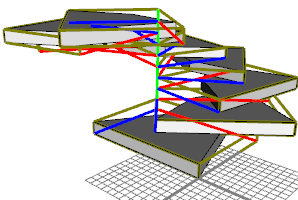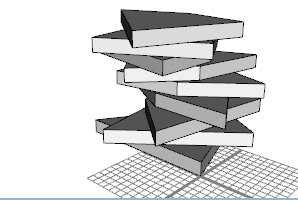# r operation

### Syntax

• r(xAngle, yAngle, zAngle)
• r(center, xAngle, yAngle, zAngle)

### Parameters

1. xAnglefloat
Angle in degrees to rotate about x- axis.
2. yAnglefloat
Angle in degrees to rotate about y- axis.
3. zAnglefloat
Angle in degrees to rotate about each z- axis.
4. centerselector
{ scopeOrgin | scopeCenter }—The rotation center. scopeOrgin is the default. (used if no center selector is given).

### Description

The r operation rotates the scope of the current shape around the pivot-axes in xyz order. The center of rotation is either the scopeOrigin (scope.t) of the current shape or the scopeCenter of the current shape.

##### Note:

r(x,y,z) is the same as rotate(rel,pivot,x,y,z)

### Examples

#### Rotation centers

 In this example, a mass model is split in vertical direction and the slices are rotated around the scope.t. Each slice's scope is shown, note how the y-axes denote an axis of symmetry.``````height = 18 dy = 2 Lot--> extrude(height) split(y) { dy : r(0, 360*split.index/split.total, 0) X }*``````The same example as above, but this time the rotations are around the scope center. ``````height = 18 dy = 2 Lot--> extrude(height) split(y) { dy : r(scopeCenter, 0, 360*split.index/split.total, 0) X }*``````